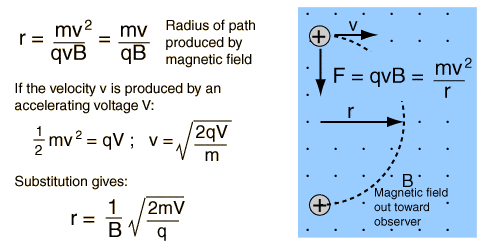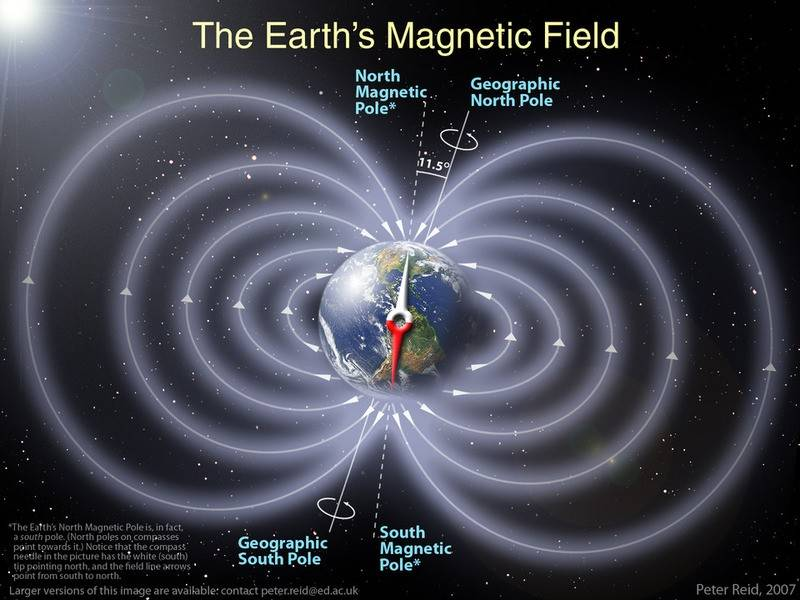# Right hand rule questions

• GaffneysWrath
In summary, the right hand rule can be used to explain how charged particles are deflected by the Earth's magnetic field. The force points in a direction perpendicular to both the direction of particle motion and the direction of the field line.f

#### GaffneysWrath

I am having difficulty using the right hand rule to explain a natural phenomenon.

Here's my concern: I know that incomming charged particles from the sun are deflected by the Earth's magnetic field because charged particles moving through a magnetic field experience a magnetic force. Here's a crude diagram to explain what I'm talking about:

Earth's Field​
^​
l​
l​
l​
SUN -----------------------------> (+) EARTH
Velocity of particle​

If you apply the right hand rule here, the resultant force points out of the screen for positively charged particles and into the screen for negative. This implies that the particles would be deflected around the Earth along the equator.

So here's my question, if the particles get deflected around the earth, how do they end up impacting the atmosphere around the poles and causing aurora's and such. How does the Earth's magnetic field direct charges towards to poles if the force doesn't point up or down? What am I missing?

Welcome to Physics Forums GaffneysWrath.When energetic charged particles enter the Earth's atmosphere from the solar wind, they tend to be channeled toward the poles by the magnetic force which causes them to spiral around the magnetic field lines of the earth.

http://hyperphysics.phy-astr.gsu.edu/hbase/atmos/aurora.html" [Broken]

Last edited by a moderator:
You're right that the direction of the force should be perpendicular to both the direction of particle motion and the direction of the field line. But don't forget that as soon as the particle's velocity changes direction as a result of this force, so too will the direction of the force change, in such a manner that the two are always perpendicular. What this means is that the particle will be drawn into circular motion in the plane perpendicular to the field line. Of course, the components of the motion parallel to the field lines remain, and since the field lines of a magnetic dipole converge at the poles, the end result is that the charged particles will SPIRAL along the field lines, traveling towards either pole in a helical motion (motion in the shape of a helix). So you see how being drawn into a circle gets rid of any of the particle's net "longitudinal" motion, leaving only a net "latitudinal" motion remaining.

. How does the Earth's magnetic field direct charges towards to poles if the force doesn't point up or down? What am I missing?

The force does point up or down.Of course, the components of the motion parallel to the field lines remain, and since the field lines of a magnetic dipole converge at the poles, the end result is that the charged particles will SPIRAL along the field lines, traveling towards either pole in a helical motion (motion in the shape of a helix). So you see how being drawn into a circle gets rid of any of the particle's net "longitudinal" motion, leaving only a net "latitudinal" motion remaining.

Thank you, excellent response. I knew there had to be a leftover component that I was neglecting. I think I see it now. I was hoping there was a really simple way of explaining it because I'm trying to find ways to make the right hand rule relevant to my students, but I think this concept is probably a little advanced for my high school seniors. I think I'll stick to mass spectrometers and particle accelerators for them. Nonetheless, I still wanted to know the answer, so thanks!